Overview

Quadratic Expressions, Equations, and Functions are found throughout the SAT, so it’s very important to be comfortable with all the key aspects of this topic. In particular, make sure you know these concepts:

• Standard Form
• Vertex Form
• Factored Form
• Properties of Parabolas
• Graphical Solutions
• Discriminant

Let’s look at each of these in more detail:

Quadratic expressions include a variable whose exponent is 2, although the product of two linear variables (for example, xy) is also quadratic. Here are some examples of quadratic expressions:Be sure you know how to translate a verbal expression into a quadratic expression like the ones shown above. Here are some examples:

 “A number times itself.”“A number multiplied by one more than the number.”“The product of a number and one less than the number.”Expressions aren’t equations but they are an important component of quadratic equations. Also, you can add and subtract quadratic expressions and still have a quadratic expression. Multiplying or dividing quadratic expressions will result in a non-quadratic expression. Here are some examples:Some of the questions you’ll be asked will involve rewriting quadratic expressions to equivalent forms. To simplify this task familiarize yourself with these quadratic identities:Binomial ExpansionBinomial SquaredDifference of Squares

Another important skill involves factoring quadratic expressions. This is a skill that also comes into play when solving quadratic equations. Here are some examples.Factoring a constant.Factoring a linear termFactoring a quadratic into a binomial squaredFactoring a quadratic into the product of two linear expressions.

 SAT Skill: Working with Quadratic Expressions   Example 1 Simplify the following expression.Combine like terms and simplify. Keep track of how the subtraction symbol will change the signs of the terms in the expression on the right.Example 2 Simplify the following expression.Expand the term on the left (use a calculator for the squared decimal terms):Combine like terms and simplify. Keep track of how the subtraction sign changes the signs of the terms on the right.Example 3 In the equation below, what is a possible value for k?You should recognize that the term on the left is a difference of squares, which can be rewritten this way:In factored form, you can find the equivalent values for k and a:Example 4 What quadratic expression is equivalent to this expression?Factor the numerator, which is a difference of squares, then simplify:Example 5 What is this expression in expanded form?Use the binomial squared identity:Example 6 Look at the following equation. If a + b = 10, what are two possible values for c?Expand the term on the left to a quadratic in standard form.Compare the two terms in standard form:From this we can conclude the following:We also know that a + b = 10. We can substitute to create this equation:Factor this quadratic to find the values for b, and corresponding values for a:Now we can find the possible values for c:Before studying what a quadratic function is, make sure you are comfortable with the following concepts, which we will also review:

• What a function is
• Independent variable
• Dependent variable
• Domain
• Range
• Different representations of functions

Brief Reviewof Functions

What Is a Function? A function is a one-to-one mapping of input values (the independent variable) to output values (the dependent variable). Click on this link to see a quick tutorial on what a function is. This slide show goes over the following key points:

• For every input value (x), there is a unique output value, f(x).
• Functions can be represented as equations, tables, and graphs.
• A function machine is a useful visual representation of the input/output nature of functions.Dependent/Independent Variables. When one variable depends on another, then it is the dependent variable. For example, the faster your speed, the farther you travel. Suppose that speed is represented by the variable s and the distance traveled is represented by the variable d

Here’s how to describe the relationship between s and d:

The faster the speed, the more distance traveled.

Distance is dependent on speed.

Distance is a function of speed.

d = f(s)

When studying functions, make sure you are comfortable telling the difference between the independent variable and dependent variable. Get comfortable using function notation. To learn more about function notation, click on this link.

Domain and Range. A function shows the relationship between two variables, the independent variable and the dependent variable. The domain is the allowed values for the independent variable. The range is the allowed values for the dependent variable. The domain and range influence what the graph of the function looks like.

For a detailed review of what domain and range are, click on this link to learn more. You’ll see definitions of the terms domain and range, as well as examples of how to find the domain and range for given functions.

Multiple Representations of Functions. We mentioned previously that functions can be represented in different ways. In fact, any function can be represented by an equation, usually f(x) equal to some expression; a table; or a graph. For a detailed review of multiple representations of functions, click on this link, to see a slide show that includes examples of these multiple representations.

The most common form of a quadratic function is the standard form.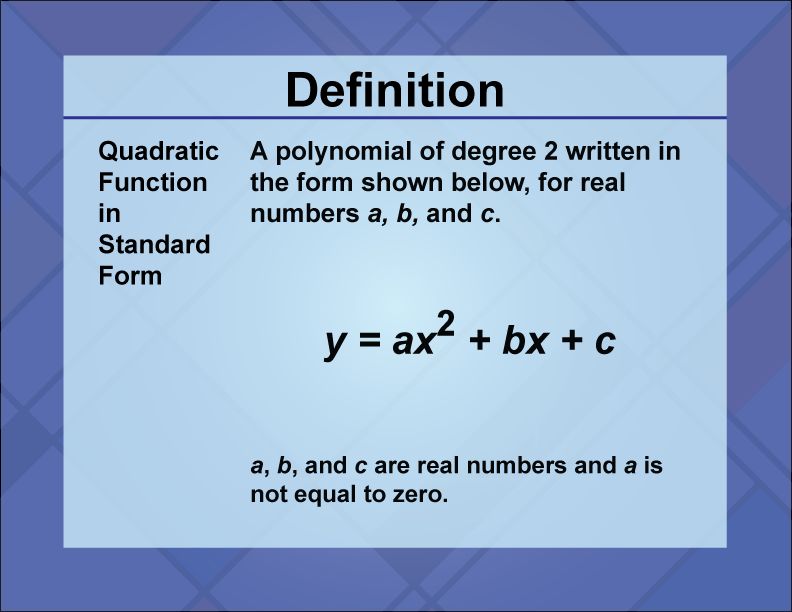The standard form is also the most common form used to solve a quadratic equation.

To see examples of graphing quadratic functions in standard form, click on this link. This slide show also includes a video tutorial.

Another way that a quadratic function can be written is in vertex form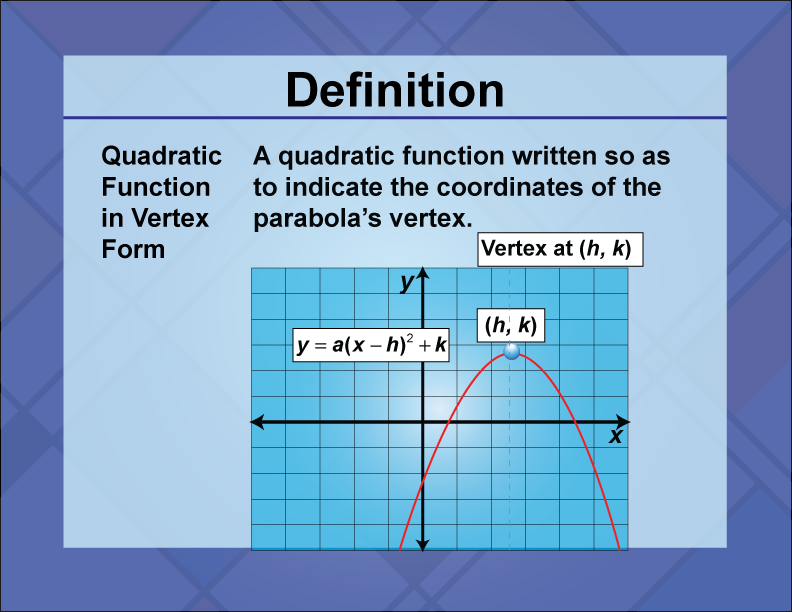To see examples of quadratic functions in vertex form, click on this link. This slide show tutorial walks you through the difference between standard form and vertex form. It also includes examples and two Desmos activities where you can graph these two types of equations.

Another way that a quadratic function can be written is in factored form. From your work with quadratic expressions, you saw some techniques for factoring a quadratic. If you can write a quadratic function as the product of linear terms, then it is much easier to solve the corresponding the quadratic equation.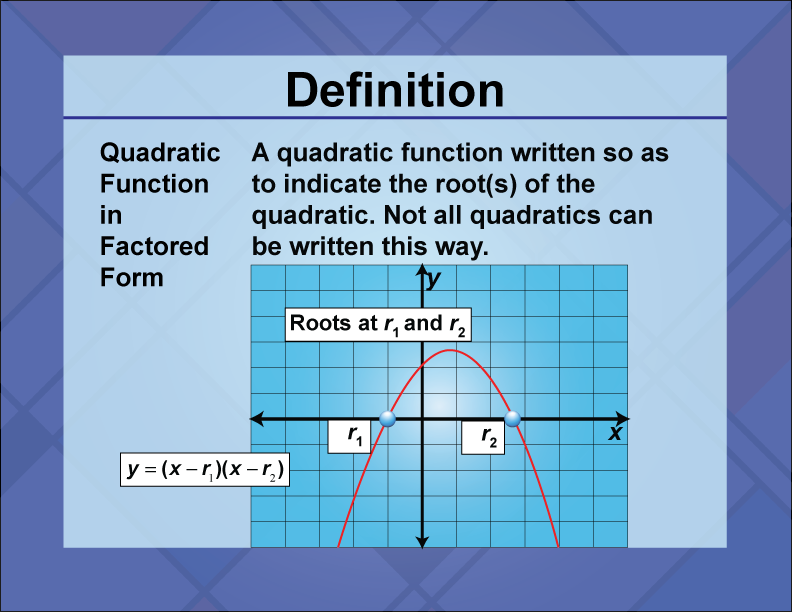To see examples of quadratic functions in factored form, click on this link

The graph of a quadratic function is known as a parabola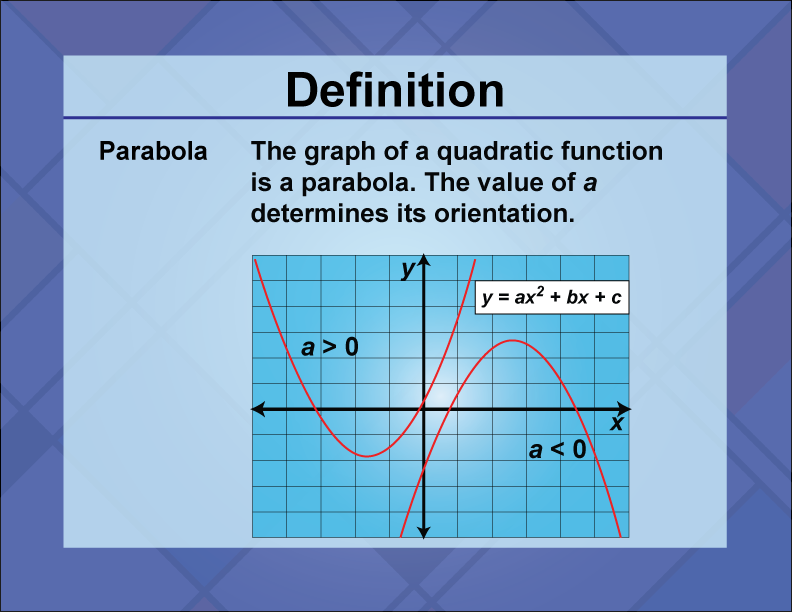Special Case of Quadratics: Equation of a Circle

The equation of a circle is not a quadratic function, but it is a quadratic relation. This is the equation of a circle:The coordinates of the center of the circle are (h, k) and the radius of the circle is r.

 SAT Skill: Working with Quadratic Functions   Example 1 In the equation below, a is a positive constant. Write the equation in factored form and write the coordinates of the vertex.A parabola in vertex form has this type of equation:Write the given equation in vertex form to find the coordinates of the vertex:Next, this equation can be written in factored form as the difference of squares (note the use of the square root sign for the non-squared term):Example 2 For the function f defined below, a is a constant and f(2) = 60. What is the value of f(5)?Use the output of f(2) to solve for a:This means that f(x) can be written this way:We can now evaluate this function for x = 5:Example 3 What is the equivalent equation of the parabola that shows the coordinates of point A?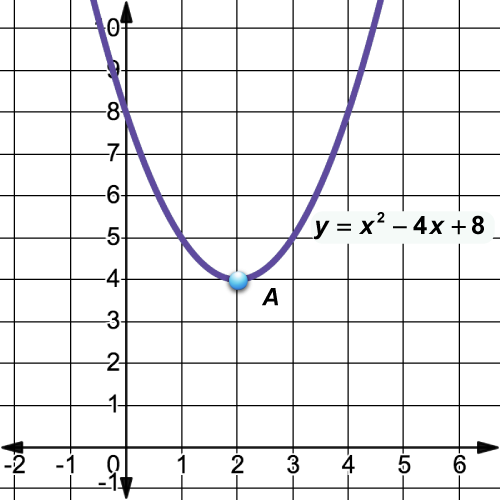The equation of the parabola shown is in standard form. Since point A of the parabola is the vertex, then the equation to write is the vertex form. Use the coordinates (2, 4) to define the vertex form:Example 4 What is the equation of a circle whose center is at (4, 5) and one of the radius endpoints is (7, 9)? The equation of the circle is of this form:We don’t know the radius of the circle yet, but we have two coordinates for a radius: the center (4, 5) and the endpoint of a radius at (7, 9). Use the distance formula to find the radius:We can now complete the equation of the circle.Example 5 A circle has this equation. What is the center of the circle?When the equation of a circle is written in standard form, the center and radius are easily found:To write the given equation in standard form, use the technique of completing the square.To learn more about the technique of completing the square, click on this link.

Quadratic equations are usually written as a quadratic expression in standard form equal to zero.A quadratic equation can have two, one, or zero real number solutions. There are several ways to solve a quadratic. These are the methods we’ll be looking at:

• Factoring

Let’s look at the first method, which will work for any quadratic equation.

When a quadratic equation is written in standard form, like the one shown below, then you can use the quadratic formula to find the solutions to the equation.Use the a, b, and c values from the quadratic equation and plug them into the quadratic formula: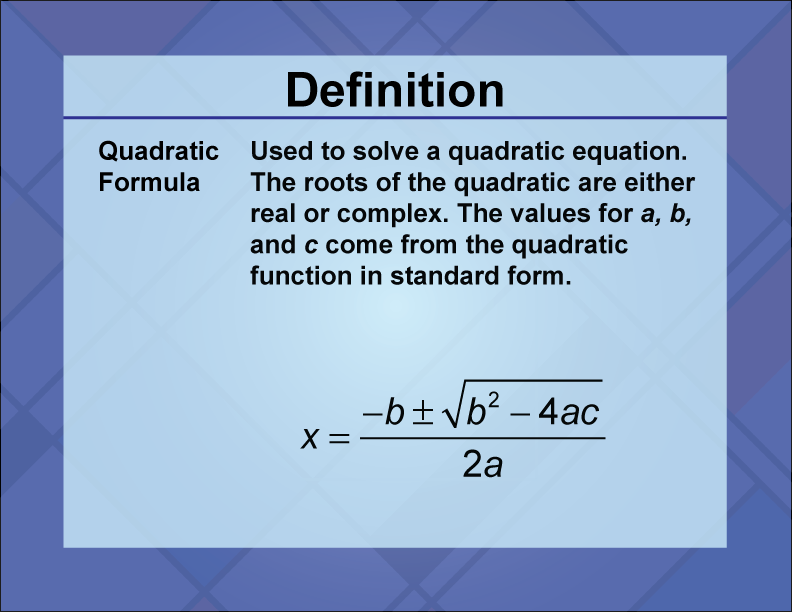Before using the quadratic formula, calculate the discriminant, which is the term under the square root sign of the quadratic formula.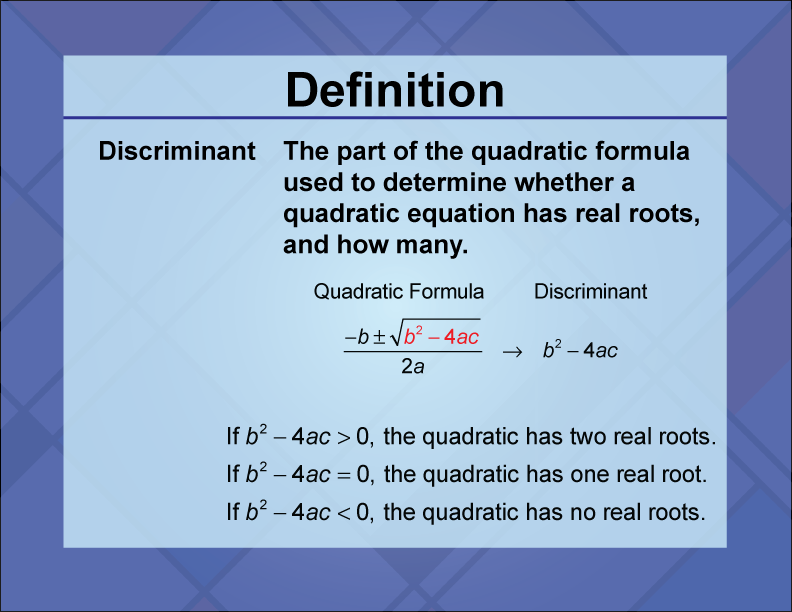To see examples of using the discriminant, click on this link.

Factoring

You’ve already seen how to factor quadratic expressions into the product of linear terms. That same idea can be used to factor certain quadratic expressions in order to find the solutions to the equation.

A factored quadratic equation will look something like this:The solutions to this equation are x = a and x = b

A more simplified version of a factored quadratic can look like this:The solutions to this equation are x = 0 and x = a

The previous two examples both had two solutions. There is a factored form that has one solution:This is the case of the binomial squared. In this case the solution to the equation is x = a

The simplest example of the binomial squared is this:The solution to this is x = 0.

If a quadratic cannot be easily factored, then you should use the quadratic formula or graph the quadratic.

To see examples of using factoring to solve a quadratic equation, click on this link.

Solving by Graphing

A visual approach to solving quadratic equations is to graph the parabola. There are three cases to look at.

Case 1: Two solutions. If the graph of the parabola intersects the x-axis twice, then there are two solutions.

Suppose you are solving this quadratic equation:To find the solution graphically, then graph the corresponding quadratic function.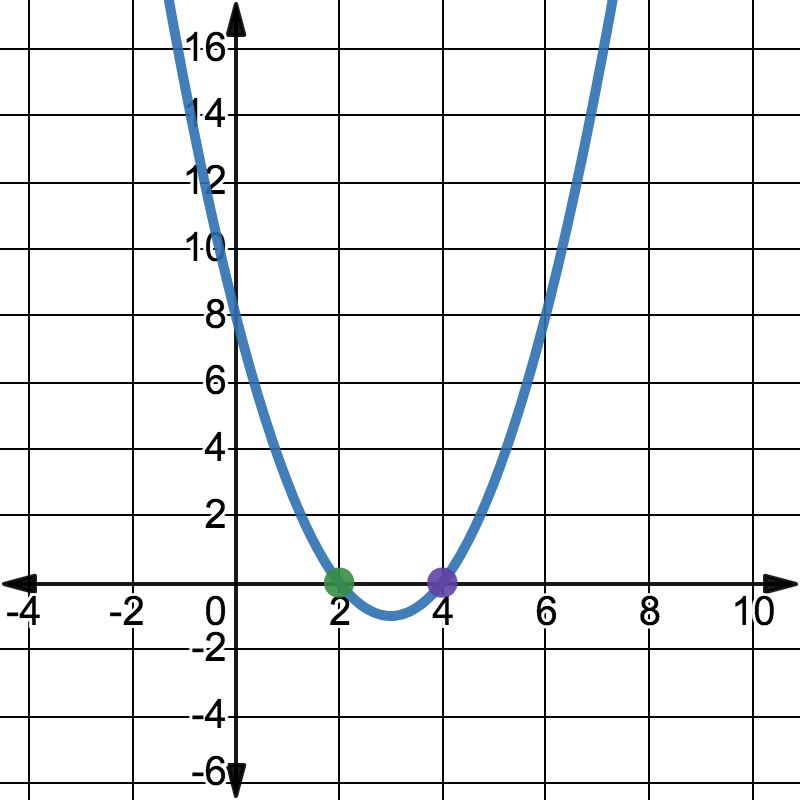Notice that this parabola intersects the x-axis at x = 2 and x = 4. Those are the solutions to the quadratic equation. In fact, you can rewrite the quadratic in factored form:Case 2: One solution. If the graph of the parabola intersects the x-axis once, then there is only one real number solution.

Suppose you are solving this quadratic equation:To find the solution graphically, then graph the corresponding quadratic function.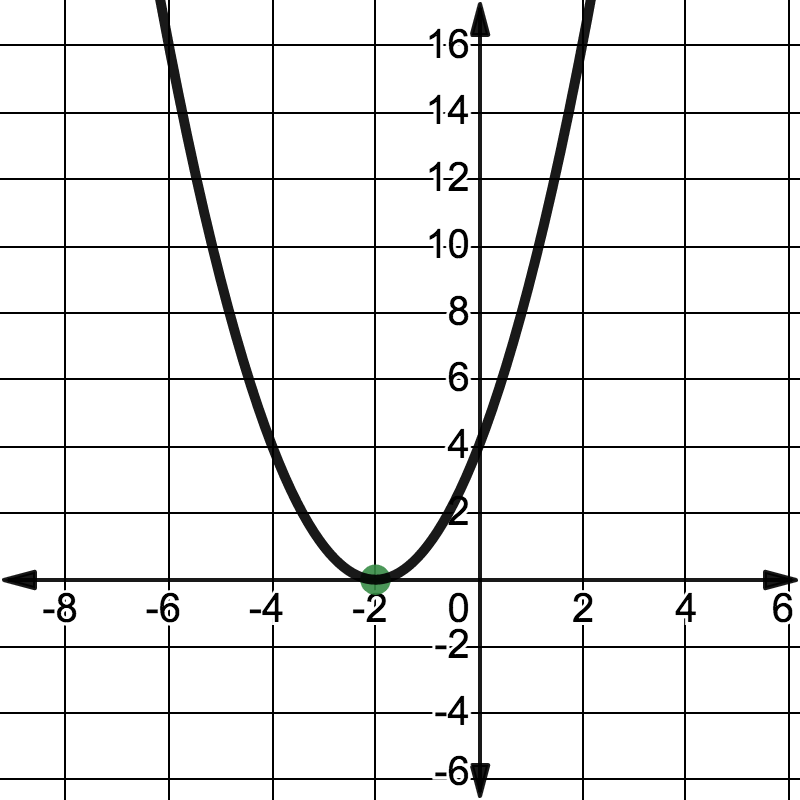Notice that this parabola intersects the x-axis at x = -2. This is the solution to the quadratic equation. In fact, you can rewrite the quadratic as a binomial squared:Case 3: No real solutions. If the graph of the parabola doesn’t intersect the x-axis, then there are no real solutions to the quadratic equation.

Suppose you are solving this quadratic equation:To find the solution graphically, then graph the corresponding quadratic function.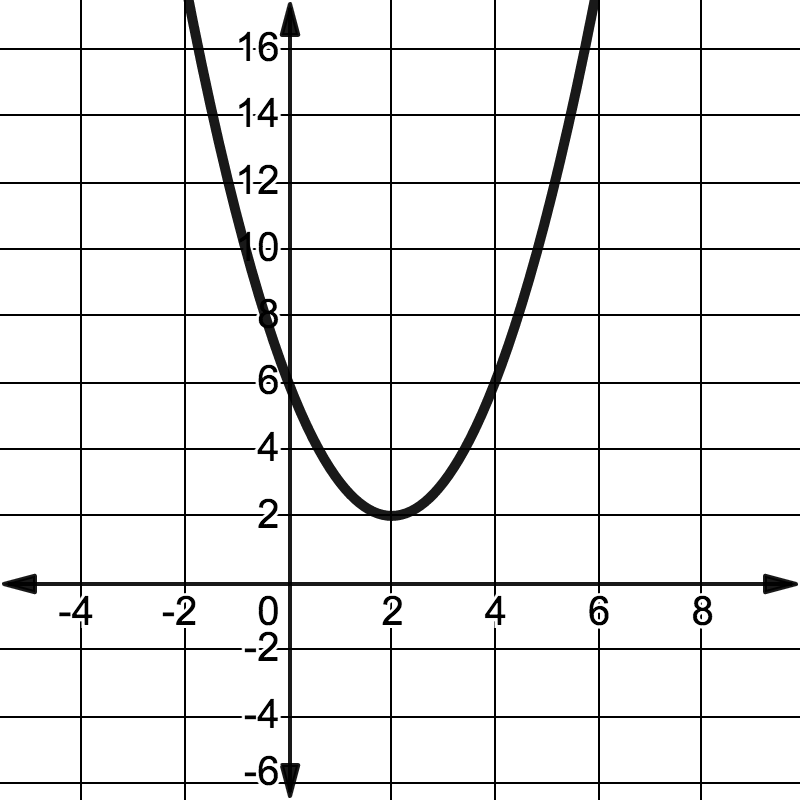Notice that this parabola doesn’t intersect the x-axis. When this happens, the quadratic equation doesn’t have real number solutions. It does, however, have complex number solutions, which you can find using the quadratic formula.

Summary of Solving by Graphing. When a parabola intersects the x-axis, then the parabola has at least one real number solution. These intersection points are also referred to as:

• X-intercepts
• Zeros of the Quadratic Function
• Roots of the Quadratic Equation

 SAT Skill: Solving Quadratic Equations Example 1 The parabola with the equation shown below intersects the line with equation y = 16 at two points, A and B. What is the length of segment AB?For a question of this type, where references are made to graphs and intersection points, it’s best to draw a diagram to get a better understanding of the problem. The equation shown is of a parabola in vertex form that intersects y = 16. Sketch that.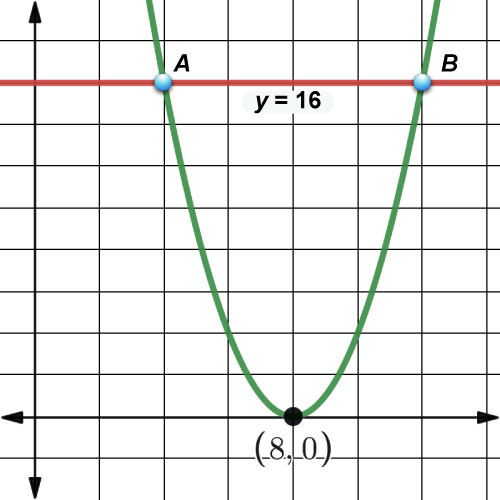We know the y coordinates for A and B; in both cases it’s 16. To find the corresponding x-coordinates, solve this equation:The x-coordinate for point A is x = 4 and the for B it’s x = 12. So the distance from A to B is the difference, or 8. Example 2 In the quadratic equation below, a is a nonzero constant. The vertex of the parabola has coordinates (c, d). Which of the following is equal to d?When a quadratic is written in standard form, the vertex has these coordinates:Write the function in standard form.Now find the corresponding x and y coordinates with this equation.Example 3 The parabola whose equation is shown below intersects the graph of y = x at (0, 0) and (a, a). What is the value of a?To get a better understanding of this problem, draw a diagram.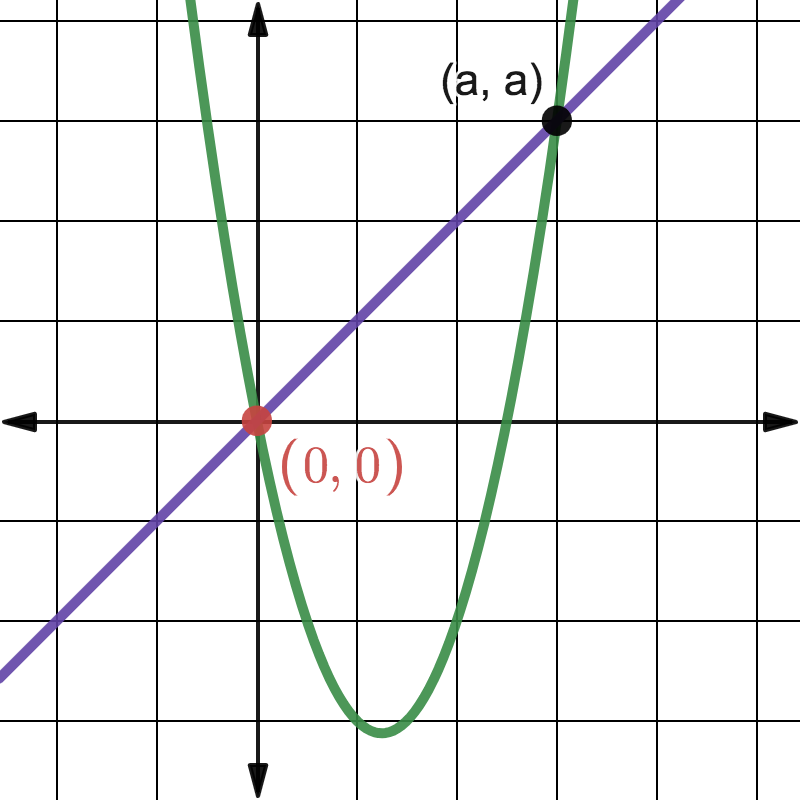To find the value of a, solve the following equation:All of the resources in this overview can be found on Media4Math. Subscribers can download these resources, or create their own slide shows using Slide Show Creator.